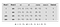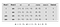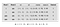# Sentence Classification in NLP

From Real-World Natural Language Processing by Masato Hagiwara

In this article, we’re going to study the task of sentence classification, where an NLP model receives a sentence and assigns some label to it.

__________________________________________________________________

Take 37% off Real-World Natural Language Processing by entering fcchagiwara into the discount code box at checkout at manning.com.
__________________________________________________________________

A spam filter is an application of sentence classification where it receives an email message and assigns whether it’s a spam or not. If you want to classify news articles into different topics (business, politics, sports, etc.), it’s also a sentence classification task. Sentence classification is one of the simplest NLP tasks that have a wide range of applications including document classification, spam filtering, and sentiment analysis. Specifically, we’re going to look at the sentiment classifier and discuss its components in detail.

## Recurrent neural networks (RNNs)

The first step in sentence classification is to represent variable-length sentences using neural networks. In this section, I’m going to present the concept of recurrent neural networks (RNNs), one of the most important concepts in deep NLP. Many modern NLP models use RNNs in some way. I’ll explain why they’re important, what they do, and introduce their simplest variant.

## Handling variable-length input

The Skip-gram network structure is simple. It takes a word vector of a fixed size, runs it through a linear layer, and obtains a distribution of scores over all the context words. The structure and the size of the input, output, and the network are all fixed throughout the training.

Many, if not most, of what we deal with in NLP are sequences of variable lengths. For example, words which are sequences of characters, can be short (“a”, “in”) or long (“internationalization”). Sentences (sequences of words) and documents (sequences of sentences) can be of any length. Even characters, if you look at them as sequences of strokes, can be simple (“O” and “L” in English) or more complex (for example, “鬱” is a Chinese character meaning “depression” which, depressingly, has twenty-nine strokes).

Neural networks can only handle numbers and arithmetic operations. This is the reason we need to convert words and documents to numbers through embeddings. Linear layers convert a fixed-length vector to another, but in order to do something similar with variable-lengths inputs, we need to figure out how to structure the neural networks to handle them.

One idea is to first convert the input (for example, a sequence of words) to embeddings, which is a sequence of vectors of floating-point numbers, and then average them. Let’s assume the input sentence is and you already know word embeddings for each word in the sentence , , etc. The average can be obtained by:

`result = (v("john") + v("loves") + v("mary") + v(".")) / 4`

This method is quite simple and it’s used in many NLP applications, but it has one critical issue, which is that it can’t take word order into account. Because the order of input elements doesn’t affect the result of averaging, you’d get the same vector for both “Mary loves John” and “John loves Mary.” Although it’s up to the task in hand, it’s hard to imagine many NLP applications wanting this kind of behavior.

Now, if we step back and reflect how we humans read language, this “averaging” is far from the reality. When we read a sentence, we don’t usually read individual words in isolation and remember them first, then move on to figuring out what the sentence means. We usually scan the sentence from the beginning, one word at a time, as we hold what the “partial” sentence means up until the part you’re reading in our short-term memory. You maintain some sort of mental representation of the sentence as you read it. When you reach the end of the sentence, the mental representation is its meaning.

Can we design a neural network structure that simulates this incremental reading of the input? The answer is a resounding yes. That structure is called Recurrent Neural Networks (RNNs), which I’ll explain in detail below.

## RNN abstraction

If you break down the reading process mentioned above, its core is the repetition of the following series of operations:

2. Based on what has been read this far (your “mental state”), figure out what the word means
3. Update the mental state
4. Move on to the next word

Let’s see how this works using a concrete example. If the input sentence is and each word is already represented as a word embedding vector. Also, let’s denote your “mental state” as , which is initialized by . Then, the reading process is represented by the following incremental operations:

`state = init_state() state = update(state, v("john")) state = update(state, v("loves")) state = update(state, v("mary")) state = update(state, v("."))`

The final value of becomes the representation of the entire sentence from this process. Notice that if you change the order in which these words are processed (for example, by flipping “John” and “Mary”), the final value of state also changes, meaning that the state also encodes some information about the word order.

You can achieve something similar if you can design a network substructure which is applied to each element of the input as it updates some internal states. RNNs are neural network structures that do exactly this. In a nutshell, an RNN is a neural network with a loop. At its core is an operation that gets applied to every element in the input as they come in. If you wrote what RNNs do in pseudo-Python, it’d be like:

`def rnn(words):     state = init_state()     for word in words:         state = update(state, word)     return state`

Notice that there’s that gets initialized first and passed around during the iteration. For every input , is updated based on the previous state and the input using the function . The network substructure corresponding to this step (the code block inside the loop) is called a cell. This stops when the input is exhausted, and the final value of becomes the result of this RNN. See figure 2 for the illustration.

Now you see the parallelism here. When you’re reading a sentence (sequence of words), your internal mental representation of the sentence, , gets updated after reading each word. You can assume that the final state encodes the representation of the entire sentence.

The only remaining work is to design two functions — and . The state is usually initialized with zero (a vector filled with zeros), and you usually don’t have to worry about how to go about defining the former. The more important issue is how you design , which determines the characteristics of the RNN.

## Simple RNN and Nonlinearity

Here, we’re going to implement , which is a function that takes two input variables and produces one output variable? After all, a cell is a neural network with its own input and output, right? The answer is yes, and it’d look like this:

`def update_simple(state, word):     return f(w1 * state + w2 * word + b)`

Notice that this is strikingly similar to the function in Section 3.4.3. In fact, if you ignore the difference in variable names, it’s exactly the same except for the function. An RNN defined by this type of the update function is called a simple RNN or Elman RNN, which, as its name suggest, one of the simplest RNN structures.

You may be wondering, then, what’s this function doing here? What does it look like? Do we need it here at all? The function, called activation function or nonlinearity, takes a single input (or a vector) and transforms it (or every element of a vector) in a non-linear fashion. Many kinds of nonlinearities play an indispensable role in making neural networks truly powerful. What they exactly do and why they’re important requires some math to understand, which is out of the scope of this article, but I’ll attempt an intuitive explanation with a simple example below.

Imagine you’re building an RNN that recognizes “grammatical” English sentences. Recognizing grammatical sentences from ungrammatical ones is a difficult NLP problem, which is a well-established research, but let’s simplify it and only consider agreement between the subject and the verb. Let’s further simplify it and assume that there are only four words in this “language” — “I”, “you”, “am”, and “are.” If the sentence is either “I am” or “you are,” it’s grammatical. Other two combinations, “I are” and “you am,” are incorrect. What you want to build is an RNN that outputs 1 for these correct sentences as it produces 0 for these incorrect ones. How would you go about building such a neural network?

The first step in almost every modern NLP model is to represent words with embeddings. Embeddings are usually learned from a large dataset of natural language text, but we’re going to give them some pre-defined values, as shown in figure 3.

Now, let’s imagine there was no activation function. The function above simplifies to:

`def update_simple_linear(state, word):     return w1 * state + w2 * word + b`

We assume the initial value of state are [0, 0], because the specific initial values aren’t relevant to the discussion here. The RNN takes the first word embedding, , updates state, takes the second word embedding, , then produces the final , which is a two-dimensional vector. Finally, the two elements in this vector are summed up and converted to . If is close to 1, the sentence is grammatical. Otherwise, it’s not. If you apply the function twice and simplify it a little bit, you get the following function, which is all this RNN does after all:

`w1 * w2 * x1 + w2 * x2 + w1 * b + b`

Remember, , , and are parameters of the model (aka “magic constants”) that need to be trained (adjusted). Here, instead of adjusting these parameters using a training dataset, let’s assign some arbitrary values and see what happens. For example, when , , and , the input and the output of this RNN is shown in figure 4.Figure 4: Input and output when w1 = [1, 0], w2 = [0, 1], and b = [0, 0] without an activation function

If you look at the values of result, this RNN groups ungrammatical sentences (for example, “I are”) with grammatical ones (for example, “you are”), which isn’t the desired behavior. How about we try another set of values for the parameters? Let’s use , , and and see what happens (figure 5).Figure 5: Input and output when w1 = [1, 0], w2 = [-1, 0], and b = [0, 0] without an activation function

This is much better, because the RNN is successful in grouping ungrammatical sentences by assigning 0 to both “I are” and “you am.” It also assigns completely opposite values (2 and -2) to grammatical sentences (“I am” and “you are”).

I’m going to stop here, but as it turns out, you can’t use this neural network to classify grammatical sentences from ungrammatical ones no matter how hard you try. No matter what values you assign to the parameters, this RNN can’t produce results that are close enough to the desired values and are able to group sentences by their grammaticality.

Let’s step back and think why this is the case. If you look at the update function above, all it does is multiply the input by some value and add them up. In a more specific term, it only transforms the input in a linear fashion. The result of this neural network always changes by some constant amount when you change the value of the input by some amount. But this is obviously not desirable — you want the result to be 1 only when the input variables are some specific values. You don’t want this RNN to be linear, you want it nonlinear.

To use an analogy, this is as if you can only use assignment (“=”), addition (“+”), and multiplication (“*”) in your programming language. You can tweak the input values to some degree to come up with the result, but you can’t write more complex logic in such a restricted setting.

Now, let’s put the activation function back and see what happens. The specific activation function we’ll use is called the hyperbolic tangent function, or more commonly, tanh, which is one of the most commonly used activation functions in neural networks. The details of this function aren’t important in this discussion, but in a nutshell, it behaves as follows: tanh doesn’t do much to the input when it’s close to zero, for example, 0.3 or -0.2. The input passes through the function almost unchanged. When the input is far from zero, tanh tries to squeeze it between -1 and 1. For example, when the input is large (say, 10.0), the output becomes close to 1.0, although it’s small (say, -10.0), the output becomes almost -1.0. This creates an effect similar to the OR logical gate (or an AND gate depending on the weights) if two or more variables are fed into the activation function. The output of the gate becomes ON (~1) and OFF (~-1) depending on the input.

When , , , and the tanh activation function is used, the result of the RNN becomes a lot closer to what we desire (see figure 6). If you round them to the closest integers, the RNN successfully groups sentence by their grammaticality.Figure 6: Input and output when w1 = [-1, 2], w2 = [-1, 2], and b = [0, 1] with an activation function

To use the same analogy, using activation functions in your neural networks is as if using ANDs and ORs and IFs in your programming language, in addition to basic math operations like additions and multiplications. In this way, you can write complex logics and model complex interactions between input variables, like this example in this section.

NOTE: The example used in this section is a slightly modified version of the popular “XOR” (or exclusive-or) example commonly seen in deep learning textbooks. This is the most basic and simplest example that can be solved by neural networks but not by other linear models.

Some final notes on RNNs — they’re trained like any other neural networks. The final outcome is compared with the desired outcome using the loss function, then the difference between the two, the loss, is used for updating the “magic constants.” The magic constants are, in this case, , , and in the function. Note that the update function and its magic constants are identical across all the timesteps in the loop. This means that what RNNs are learning is a general form of updates that can be applied to any situation.

That’s all for now.

If you want to learn more about the book, check it out on our browser-based liveBook reader here and see this slide deck.